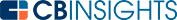# What is a Weighted Average?

A weighted average assigns importance to values before calculating their average. This importance is represented mathematically by multiplying each value by its own predetermined "weight" number.

## Why use a weighted average?

Weighted averages can generate more accurate results than simple averages produce. Weight can account for factors such as frequency, quantity, intensity, and more. Unlike simple averages that weigh all input values equally, a weighted average gives greater importance to some values than others, basing the calculated weight on other real-world factors.

When predetermined weights don’t add up to 1, multiply all values by their weights and add these together. Then, divide this number by the sum of all of the weights to obtain the weighted average.

## Weighted average use cases

Weighted average calculations can help calculate collective figures that account for the differences between their constituent values. This makes weighted averages useful for purposes such as the following:

• Weighted average returns — This kind of weighted average helps with estimating a company's returns over a given period of time. In weighted average returns, the weight values should add up to 100% and can be calculated by dividing each value by the sum of all of the values together.
• Weighted average cost of capital — This weighted average figure shows how much the operating funds a company utilizes ultimately cost.
• Volume weighted average price — This weighted average is used to track a security's price fluctuations and assign an average price to these over a given time period. By weighting the price of a security by the volume of orders that have gone through at such a price, you can create an accurate portrayal of the security's performance.
• Weighted average market capitalization — This figure is often used to create more accurate indicators of a stock market's performance. A prominent example of this would be the S&P 500.

Essentially, a weighted average is a calculation used for a variety of purposes when simple averages are insufficient to account for differences in the amount of influence various factors have on an outcome

You may also like# www.cbinsights.com